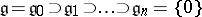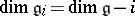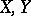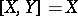# Lie algebra, supersolvable

(diff) ← Older revision | Latest revision (diff) | Newer revision → (diff)

triangular Lie algebra

A finite-dimensional Lie algebraover a fieldfor which the eigen values of the operatorsof the adjoint representation belong tofor all(cf. Adjoint representation of a Lie group).

A supersolvable Lie algebra is solvable. The class of supersolvable Lie algebras contains the class of nilpotent Lie algebras and is contained in the class of exponential Lie algebras (cf. Lie algebra, nilpotent; Lie algebra, exponential). It is closed with respect to transition to subalgebras, quotient algebras and finite direct sums, but it is not closed with respect to extensions.

A supersolvable Lie algebra over a perfect field has many of the properties of solvable Lie algebras (cf. Lie algebra, solvable) over an algebraically closed field (Lie's theorem, the presence of a chain of idealsfor which, and others). In an arbitrary finite-dimensional Lie algebrathere are maximal supersolvable subalgebras and they contain the nil radical. Ifor, or ifis perfect andis an algebraic linear Lie algebra, then all supersolvable subalgebras are conjugate. The Lie algebraovercorresponding to a-split algebraic group (cf. Split group) over a perfect fieldis a supersolvable Lie algebra.

Any supersolvable Lie algebra over a field of characteristic 0 can be isomorphically imbedded in the Lie algebra of upper-triangular matrices with coefficients from(which is itself supersolvable). The simplest example of a supersolvable Lie algebra that is not nilpotent is a two-dimensional Lie algebra with basisand defining relation. For references see Lie group, supersolvable.

How to Cite This Entry:
Lie algebra, supersolvable. Encyclopedia of Mathematics. URL: http://encyclopediaofmath.org/index.php?title=Lie_algebra,_supersolvable&oldid=17913
This article was adapted from an original article by V.V. Gorbatsevich (originator), which appeared in Encyclopedia of Mathematics - ISBN 1402006098. See original article